#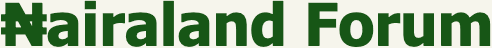Welcome, Guest: Join Nairaland / LOGIN! / Trending / Recent / New
Stats: 2,759,180 members, 6,559,506 topics. Date: Wednesday, 27 October 2021 at 09:41 PM

## Maths Guru Please Help Me Solve This Simultaneous Equations For My Young(photo) - Education - Nairaland

 Maths Guru Please Help Me Solve This Simultaneous Equations For My Young(photo) by Sirgay: 7:20pm On Nov 26, 2017 please, I will be waiting for the solution. Wasn't good in maths. elimination of substitution method 1 Like 1 Share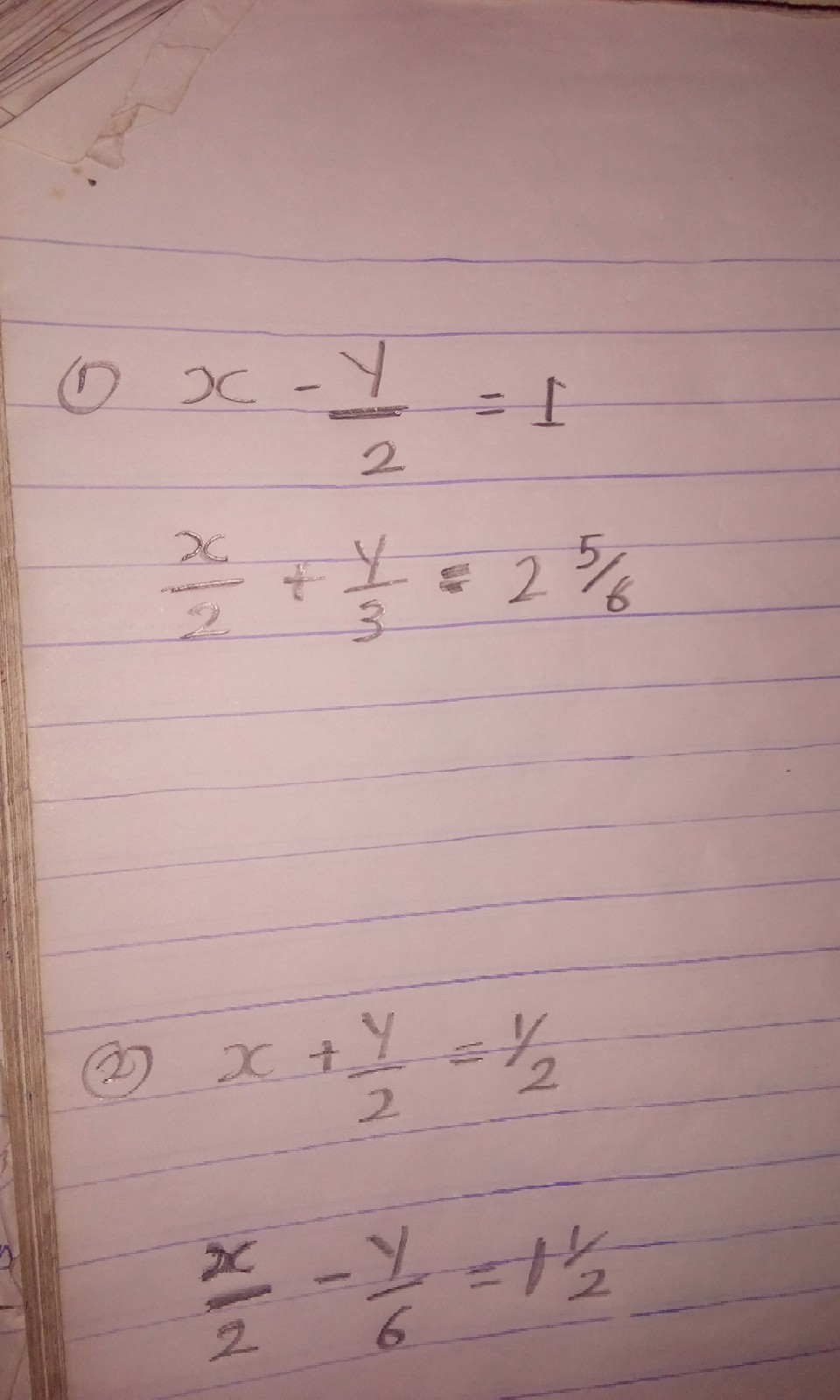Re: Maths Guru Please Help Me Solve This Simultaneous Equations For My Young(photo) by Swissheart(f): 7:23pm On Nov 26, 2017 naturalwaves see this Re: Maths Guru Please Help Me Solve This Simultaneous Equations For My Young(photo) by AJOBI77(m): 7:26pm On Nov 26, 2017 Use substitution method Re: Maths Guru Please Help Me Solve This Simultaneous Equations For My Young(photo) by Sirgay: 7:28pm On Nov 26, 2017 Swissheart:naturalwaves see this Thanks a lot I appreciate, she is submitting tomorrow. The questions are 5. 1 Share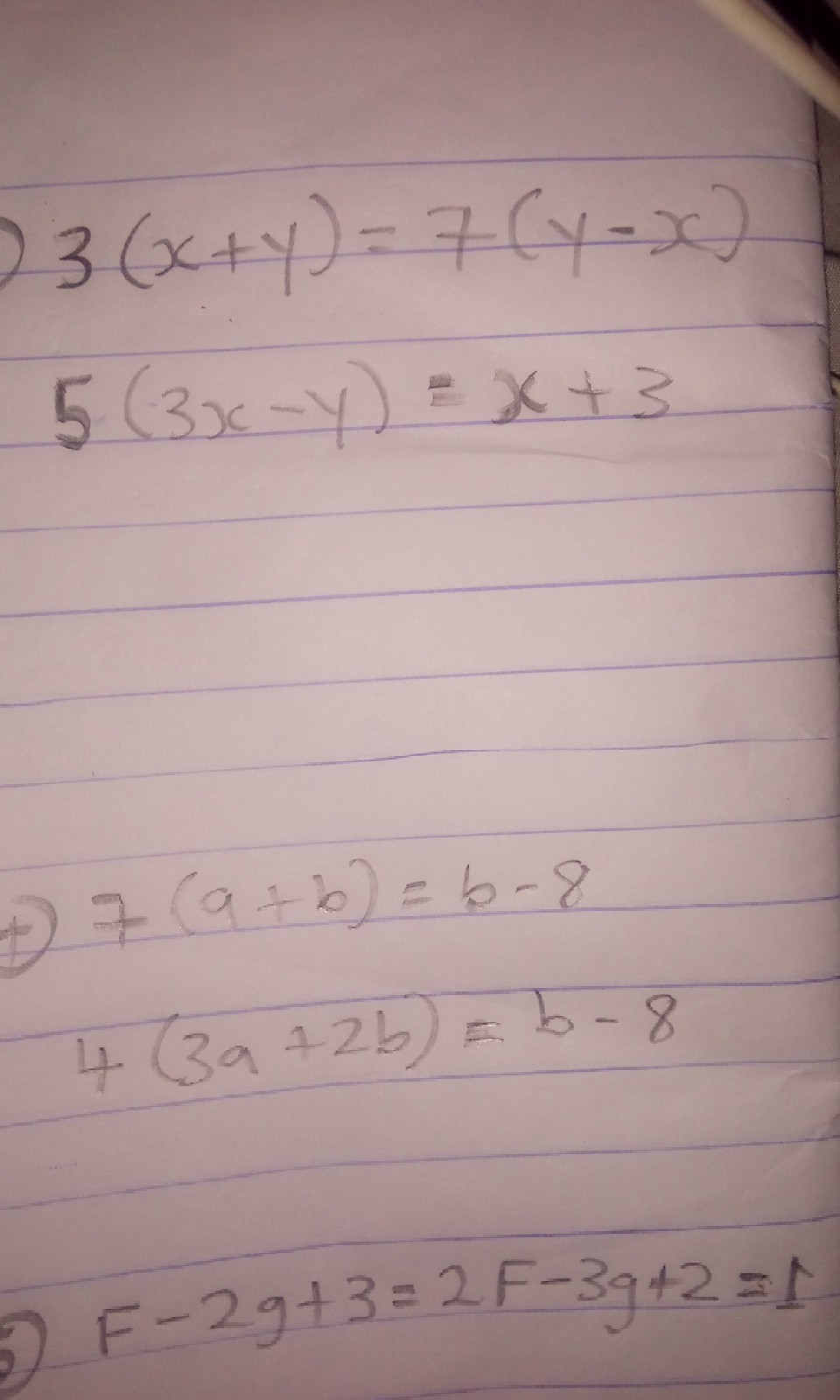Re: Maths Guru Please Help Me Solve This Simultaneous Equations For My Young(photo) by Sirgay: 7:29pm On Nov 26, 2017 AJOBI77:Use substitution method Bros I don't know, please help or mention someone who can, this must be solved this night. Re: Maths Guru Please Help Me Solve This Simultaneous Equations For My Young(photo) by Sirgay: 7:32pm On Nov 26, 2017 cc fynestboi Re: Maths Guru Please Help Me Solve This Simultaneous Equations For My Young(photo) by generalbush(m): 7:37pm On Nov 26, 2017 for Number 5SolutionF - 2g = -32f - 3g = 1-2Using Elimination MethodMutiply eqn 1 by 2 and eqn 2 by 1=> F - 2g = -3 ----- x 2 2F - 3g = -1 ------ x 1This will give us2F - 4g = -6 2F - 3g = -1Subtract eqn 2 from eqn 1==> -g = - 5divide both sides by -==> g = 5Substitute g = 5 in eqn 12F - 4 (5) = -62F - 20 = -62F = -6 + 202F = 14F = 14/2F = 7.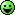FOR QUESTION 4Typing... 4 Likes Re: Maths Guru Please Help Me Solve This Simultaneous Equations For My Young(photo) by Sirgay: 7:38pm On Nov 26, 2017 generalbush:for Number 5 Solution F - 2g = 13 2f - 3g = 1-2 Using Elimination Method Mutiply eqn 1 by 2 and eqn 2 by 1 => F - 2g = -3 ----- x 2 2F - 3g = -1 ------ x 1 This will give us 2F - 4g = -6 2F - 3g = -1 Subtract eqn 2 from eqn 1 ==> -g = 5 Substitute g = 5 in eqn 1 2F - 4 (5) = -6 2F - 20 = -6 2F = -6 + 20 2F = 14 F = 14/2 F = 7.You didn't get the question right. please check again. Re: Maths Guru Please Help Me Solve This Simultaneous Equations For My Young(photo) by generalbush(m): 7:45pm On Nov 26, 2017 Sirgay: You didn't get the question right. please check again.But I already cross checked. It gave me a balanced equation. Re: Maths Guru Please Help Me Solve This Simultaneous Equations For My Young(photo) by Sirgay: 7:47pm On Nov 26, 2017 generalbush:But I already cross checked. It gave me a balanced equation. There was no 13 in the question, please check again. Re: Maths Guru Please Help Me Solve This Simultaneous Equations For My Young(photo) by generalbush(m): 7:49pm On Nov 26, 2017 Sirgay: There was no 13 in the question, please check again.Mistake.I wanted to type "-3"I will edit the question.Re: Maths Guru Please Help Me Solve This Simultaneous Equations For My Young(photo) by Sirgay: 7:50pm On Nov 26, 2017 generalbush: Mistake.I wanted to type "-3" I will edit the question.Ok please carry on Re: Maths Guru Please Help Me Solve This Simultaneous Equations For My Young(photo) by generalbush(m): 7:57pm On Nov 26, 2017 Sirgay: Ok please carry onFor question 1[s][/s]X/1 - Y/2 = 1 -------------- eqn 1Removing equations, we will multiply all sides by 22(x/1) - 2(y/2) = 2 * 1This will give us...2x - y = 2 ----- Eqn 1Solving eqn 2...x/2 + y/3 = 17/6Multiplying both sides by 6, it gives us...6 * x/2 + 6* y/3 = 6 * 17/6==> 3x + 2y = 17 ---- Eqn 2Combining both equations...2x - y = 23x + 2y = 17Using Elimination method, I multiply eqn 1 by 2 and eqn 1 by 1It gives me ==> 2* 2x - 2*y = 2*2 1* 3x + 1* 2y = 1* 17It gives us...4x - 2y = 43x + 2y = 17Subtracting eqn 2 from 1It gives us...7x = 22x= 22/7Substituting x = 22/7 in eqn 1, we have2 (22/7) - y = 244/7 - y = 2Multiply all sides by 77* 44/7 - 7*y = 7*2==> 20 -7y = 14- 7y = 14 - 44 -7y = -30Divide both sides by -y = 30/7 1 Like Re: Maths Guru Please Help Me Solve This Simultaneous Equations For My Young(photo) by Sirgay: 8:11pm On Nov 26, 2017 generalbush: For question 1[s][/s] X/1 - Y/2 = 1 -------------- eqn 1 Removing equations, we will multiply all sides by 2 2(x/1) - 2(y/2) = 2 * 1 This will give us... 2x - y = 2 ----- Eqn 1 Solving eqn 2... x/2 + y/3 = 60/6 Multiplying both sides by 6, it gives us... 6 * x/2 + 6* y/3 = 6 * 60/6 ==> 3x + 2y = 60 ---- Eqn 2 Combining both equations... 2x - y = 2 3x + 2y = 60 Using Elimination method, I multiply eqn 1 by 2 and eqn 1 by 1 It gives me ==> 2* 2x - 2*y = 2*2 1* 3x + 1* 2y = 1*6 It gives us... 4x - 2y = 4 3x + 2y = 6 Subtracting eqn 2 from 1 It gives us... 7x = 10 x= 10/7 Substituting x = 10/7 in eqn 1, we have 2 (10/7) - y = 2 20/7 - y = 2 Multiply all sides by 7 7* 20/7 - 7*y = 7*2 ==> 20 -7y = 14 - 7y = 14 - 20 -7y = -6 Divide both sides by - y = 6/7 Thanks generalbush, I can't thank you more about 1 Like 1 Share Re: Maths Guru Please Help Me Solve This Simultaneous Equations For My Young(photo) by Sirgay: 8:15pm On Nov 26, 2017 generalbush please check how you got 60/6 (60 over 6) the improper fraction or something, isn't there an error? Re: Maths Guru Please Help Me Solve This Simultaneous Equations For My Young(photo) by dejt4u(m): 8:19pm On Nov 26, 2017 Sirgay:please, I will be waiting for the solution. Wasn't good in maths. elimination of substitution methodchange all the equation to non-fractional linear equation for question 1 and 2. for question 1, multiply eqn (i) by 2 and eqn (ii) by 6for question 2, multiply eqn (i) by 2 and eqn (ii) by 6 Re: Maths Guru Please Help Me Solve This Simultaneous Equations For My Young(photo) by Sirgay: 8:20pm On Nov 26, 2017 dejt4u:change all the equation to non-fractional linear equation for question 1 and 2. for question 1, multiply eqn (i) by 2 and eqn (ii) by 6for question 2, multiply eqn (i) by 2 and eqn (ii) by 6 please help me with that. I can't am too old for maths lesson. Re: Maths Guru Please Help Me Solve This Simultaneous Equations For My Young(photo) by Sirgay: 8:27pm On Nov 26, 2017 generalbush will 2 whole number 5 0ver 6 gives us 60 over 6 in that question one solution? please check. Re: Maths Guru Please Help Me Solve This Simultaneous Equations For My Young(photo) by generalbush(m): 8:28pm On Nov 26, 2017 Sirgay:generalbush will 2 whole number 5 0ver 6 gives us 60 over 6 in that question one solution? please check. Nop. Re: Maths Guru Please Help Me Solve This Simultaneous Equations For My Young(photo) by Sirgay: 8:29pm On Nov 26, 2017 generalbush: Nop. I know that must be an error, thanks for confirming it. Re: Maths Guru Please Help Me Solve This Simultaneous Equations For My Young(photo) by priceaction: 8:30pm On Nov 26, 2017 Just download photomath from play store and scan ( via your phone camera) or type your questions then safe your self the stress of coming here. Life has been made easier in this generation. 1 Like Re: Maths Guru Please Help Me Solve This Simultaneous Equations For My Young(photo) by generalbush(m): 8:34pm On Nov 26, 2017 Sirgay: I know that must be an error, thanks for confirming it.I will edit now.I have edited it Re: Maths Guru Please Help Me Solve This Simultaneous Equations For My Young(photo) by generalbush(m): 8:38pm On Nov 26, 2017 Sirgay: I know that must be an error, thanks for confirming it.Please edit the wrong answers you quoted. So that it won't confuse Op Re: Maths Guru Please Help Me Solve This Simultaneous Equations For My Young(photo) by Sirgay: 8:42pm On Nov 26, 2017 generalbush: Please edit the wrong answers you quoted. So that it won't confuse Op Ok Re: Maths Guru Please Help Me Solve This Simultaneous Equations For My Young(photo) by Yemluvkid(m): 8:52pm On Nov 26, 2017 generalbush:for Number 5SolutionF - 2g = -32f - 3g = 1-2Using Elimination MethodMutiply eqn 1 by 2 and eqn 2 by 1=> F - 2g = -3 ----- x 2 2F - 3g = -1 ------ x 1This will give us2F - 4g = -6 2F - 3g = -1Subtract eqn 2 from eqn 1==> -g = - 5divide both sides by -==> g = 5Substitute g = 5 in eqn 12F - 4 (5) = -62F - 20 = -62F = -6 + 202F = 14F = 14/2F = 7.FOR QUESTION 4Typing...This is wrong. You supposed to equate both the first and second terms to 1. Like... F - 2g +3= 12f - 3g +2= 1Which will gives F-2g=-2...........(1)2f-3g=-1...........(2)Multiplying (1) by 2 gives 2f-4g=-4.......(3)2f-3g=-1.......(2)Subtracting eqn (2) from the (3) gives -g=-3g=3Substitute for g in equation 1F-2g=-2F-2(3)=-2F-6=-2F=-2+6F=4 Re: Maths Guru Please Help Me Solve This Simultaneous Equations For My Young(photo) by Deicide: 9:01pm On Nov 26, 2017 generalbush: For question 1[s][/s] X/1 - Y/2 = 1 -------------- eqn 1 Removing equations, we will multiply all sides by 2 2(x/1) - 2(y/2) = 2 * 1 This will give us... 2x - y = 2 ----- Eqn 1 Solving eqn 2... x/2 + y/3 = 17/6 Multiplying both sides by 6, it gives us... 6 * x/2 + 6* y/3 = 6 * 17/6 ==> 3x + 2y = 17 ---- Eqn 2 Combining both equations... 2x - y = 2 3x + 2y = 17 Using Elimination method, I multiply eqn 1 by 2 and eqn 1 by 1 It gives me ==> 2* 2x - 2*y = 2*2 1* 3x + 1* 2y = 1* 17 It gives us... 4x - 2y = 4 3x + 2y = 17 Subtracting eqn 2 from 1 It gives us... 7x = 22 x= 22/7 Substituting x = 22/7 in eqn 1, we have 2 (22/7) - y = 2 44/7 - y = 2 Multiply all sides by 7 7* 44/7 - 7*y = 7*2 ==> 20 -7y = 14 - 7y = 14 - 44 -7y = -30 Divide both sides by - y = 30/7 You are wrong the answer is Y = 4 X = 3 Re: Maths Guru Please Help Me Solve This Simultaneous Equations For My Young(photo) by dejt4u(m): 9:01pm On Nov 26, 2017 Sirgay: OkSolution to Q1Edited.. Answer to Q1 is x = 3 and y = 4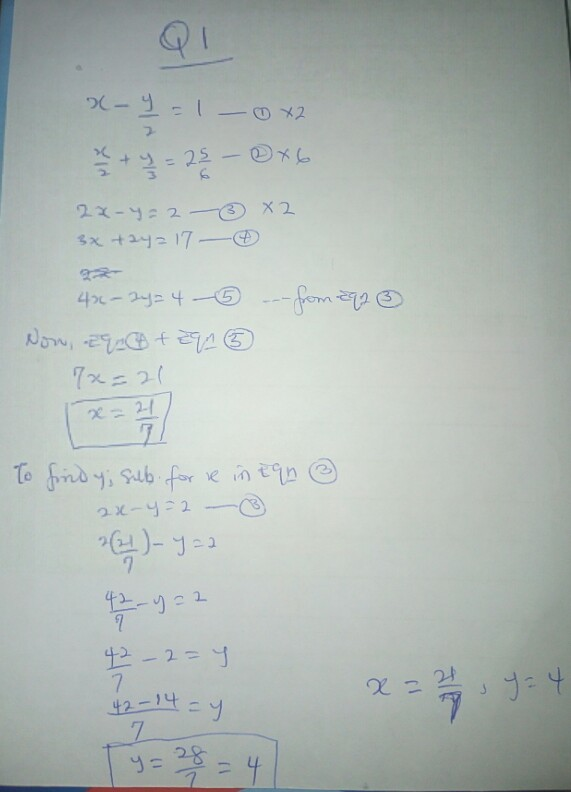Re: Maths Guru Please Help Me Solve This Simultaneous Equations For My Young(photo) by dejt4u(m): 9:03pm On Nov 26, 2017 Answer to Question 2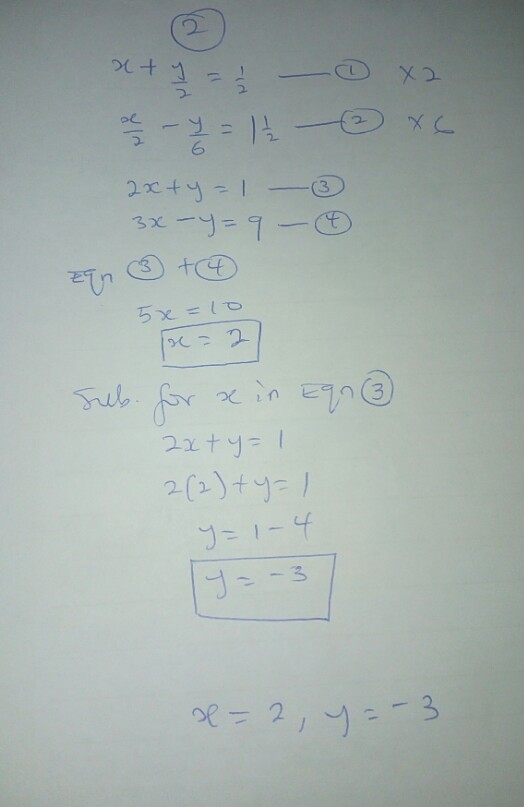Re: Maths Guru Please Help Me Solve This Simultaneous Equations For My Young(photo) by Yemluvkid(m): 9:04pm On Nov 26, 2017 ... Re: Maths Guru Please Help Me Solve This Simultaneous Equations For My Young(photo) by dejt4u(m): 9:04pm On Nov 26, 2017 Solution to Question 3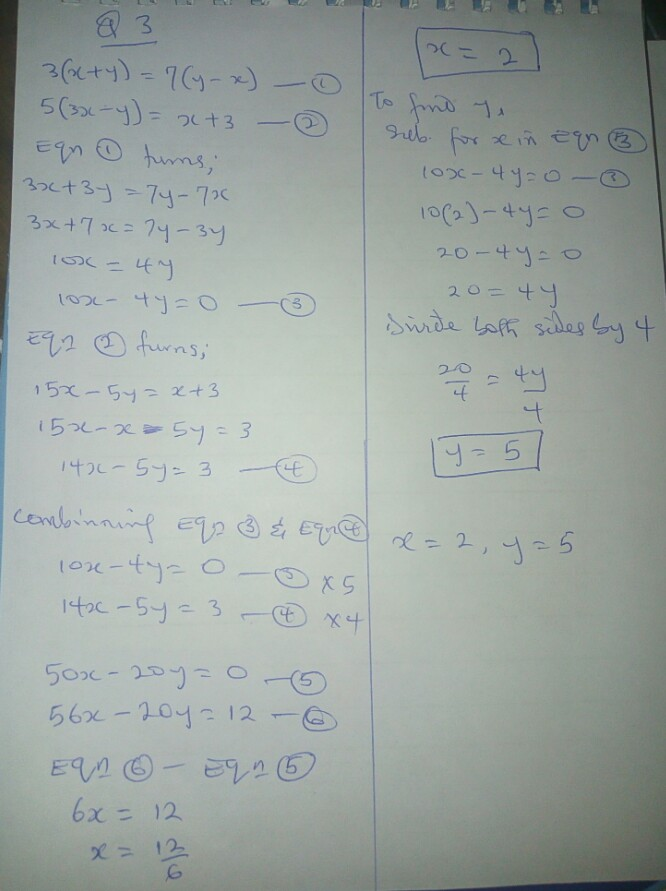Re: Maths Guru Please Help Me Solve This Simultaneous Equations For My Young(photo) by dejt4u(m): 9:05pm On Nov 26, 2017 Solution to Question 4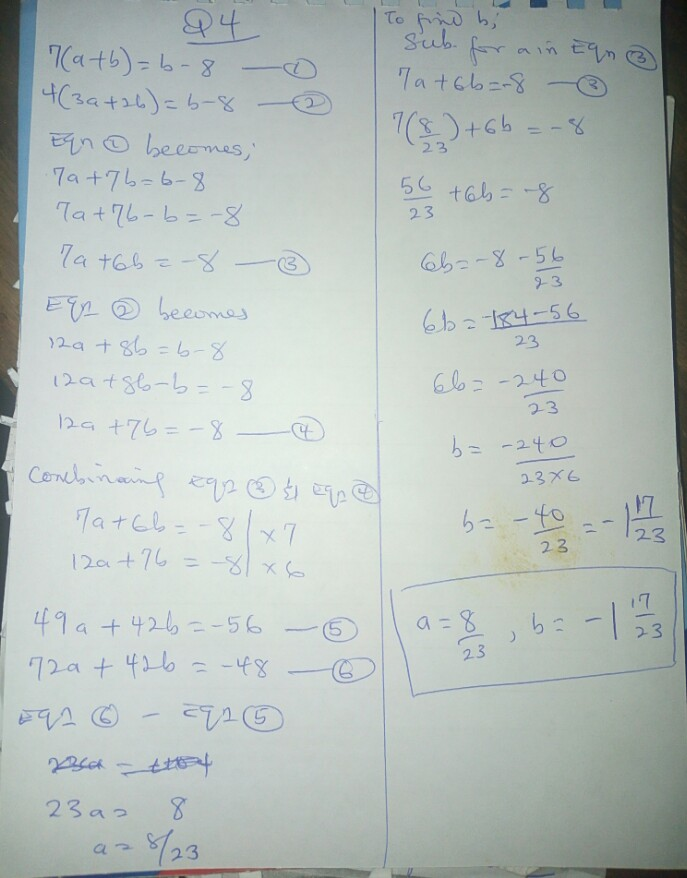Re: Maths Guru Please Help Me Solve This Simultaneous Equations For My Young(photo) by Yemluvkid(m): 9:05pm On Nov 26, 2017 [/quote][/color]generalbush: For question 1[s][/s] X/1 - Y/2 = 1 -------------- eqn 1 Removing equations, we will multiply all sides by 2 2(x/1) - 2(y/2) = 2 * 1 This will give us... 2x - y = 2 ----- Eqn 1 Solving eqn 2... x/2 + y/3 = 17/6 Multiplying both sides by 6, it gives us... 6 * x/2 + 6* y/3 = 6 * 17/6 ==> 3x + 2y = 17 ---- Eqn 2 Combining both equations... 2x - y = 2 3x + 2y = 17 Using Elimination method, I multiply eqn 1 by 2 and eqn 1 by 1 It gives me ==> 2* 2x - 2*y = 2*2 1* 3x + 1* 2y = 1* 17 It gives us... 4x - 2y = 4 3x + 2y = 17 Subtracting eqn 2 from 1 It gives us... 7x = 22 x= 22/7 Substituting x = 22/7 in eqn 1, we have 2 (22/7) - y = 2 44/7 - y = 2 Multiply all sides by 7 7* 44/7 - 7*y = 7*2 ==> 20 -7y = 14 - 7y = 14 - 44 -7y = -30 Divide both sides by - y = 30/7 Check the solution again 17+4 is 21... Therefore x=7